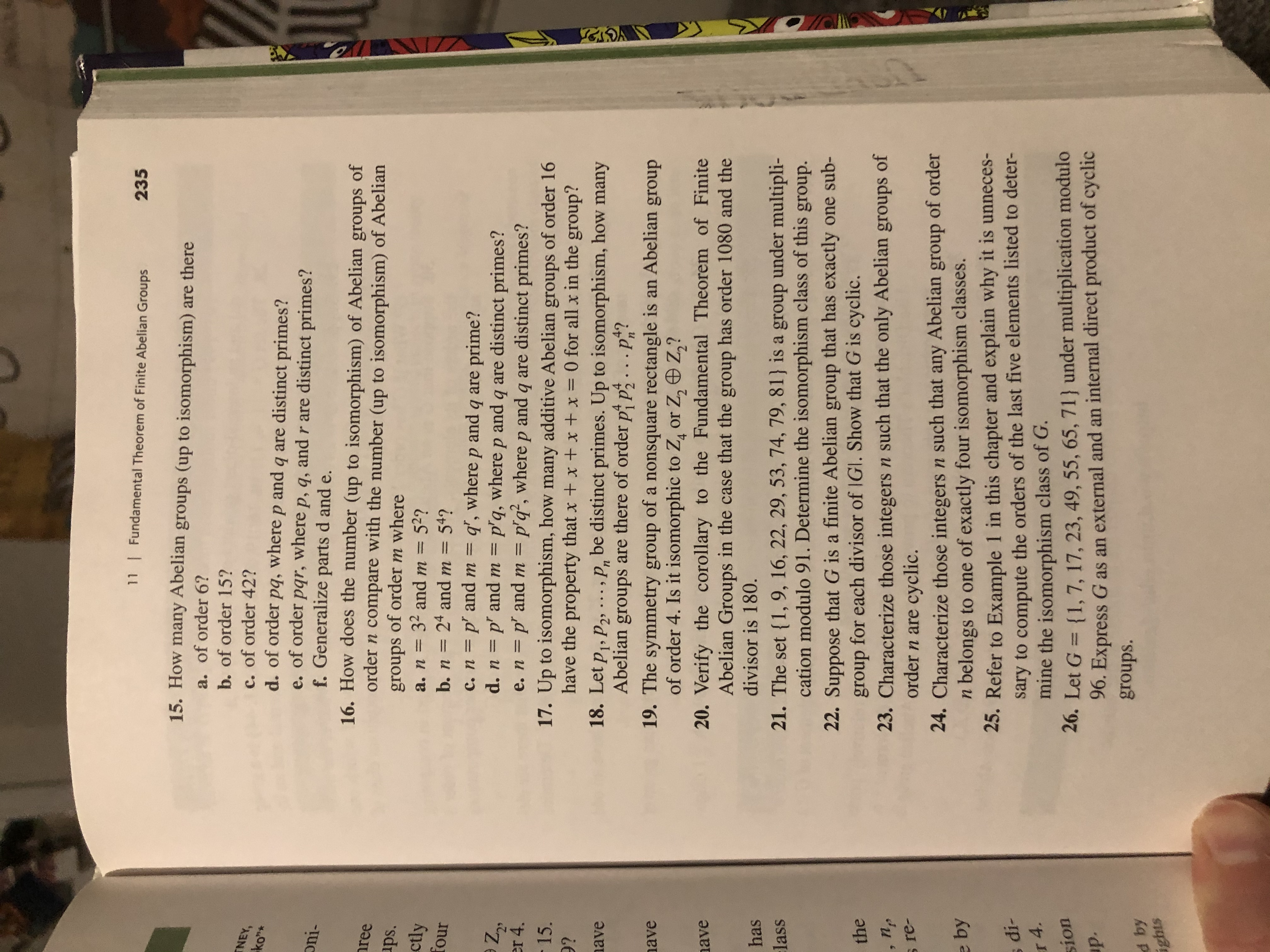# 11Fundamental Theorem of Finite Abelian Groups23515. How many Abeliangroups (up to isomorphism) are therea. of order 6?b. of order 15?NEY,c. of order 42?ko"*d. of order pq, where p andof order par, wheref. Generalize parts d andq are distinct primes?P, q, and r are distinct primes?e.oni-16. How does the number (up to isomorphism) of Abelianofreegroupsorder n compare with the number (up to isomorphism) of Abelianups.groups of order m where32 and m 52?24 and m 54?ctlyа. п —fourb. nc. n p' and m = q', where p and q are prime?d. n p andm = p'q, where p and q are distinct primes?e. n p' and m = p'q', where p and q are distinct primes?17. Up to isomorphism, how many additive Abelian groups of order 16have the property that x + x +x + x = 0 for all x in the group?er 4.- 15.9?ave18. Let pi, P2 .. , be distinct primes. Up to isomorphism, how manyAbelian groups are there of order p p...p?ave19. The symmetry group of a nonsquare rectangle is an Abelian groupof order 4. Is it isomorphic to Zg or Z, Z,?420. Verify the corollary to the Fundamental Theorem of FiniteAbelian Groups in the case that the group has order 1080 and theavedivisor is 180.has{1,9, 16, 22, 29, 53, 74, 79, 81 is a group under multipli-cation modulo 91. Determine the isomorphism class of this group.:lass21. The set22. Suppose that G is a finite Abelian group that has exactly one sub-group for each divisor of IGl. Show that G is cyclic.23. Characterize those integers n such that the only Abelian groups oforder n are cyclic.the, npre-24. Characterize those integersn such that any Abelian group of ordern belongs to one of exactly four isomorphism classes.25. Refer to Example 1 in this chapter and explain why it is unneces-sary to compute the orders of the last five elements listed to deter-mine the isomorphism class of G.26. Let G {1,7, 17, 23, 49, 55, 65, 71} under multiplication modulo96. Express G as an external and an internal direct product of cyclice bydi-r4sionр.groups.d byights

Question
2 views

26help_outlineImage Transcriptionclose11 Fundamental Theorem of Finite Abelian Groups 235 15. How many Abelian groups (up to isomorphism) are there a. of order 6? b. of order 15? NEY, c. of order 42? ko"* d. of order pq, where p and of order par, where f. Generalize parts d and q are distinct primes? P, q, and r are distinct primes? e. oni- 16. How does the number (up to isomorphism) of Abelian of ree groups order n compare with the number (up to isomorphism) of Abelian ups. groups of order m where 32 and m 52? 24 and m 54? ctly а. п — four b. n c. n p' and m = q', where p and q are prime? d. n p andm = p'q, where p and q are distinct primes? e. n p' and m = p'q', where p and q are distinct primes? 17. Up to isomorphism, how many additive Abelian groups of order 16 have the property that x + x +x + x = 0 for all x in the group? er 4. - 15. 9? ave 18. Let pi, P2 .. , be distinct primes. Up to isomorphism, how many Abelian groups are there of order p p...p? ave 19. The symmetry group of a nonsquare rectangle is an Abelian group of order 4. Is it isomorphic to Zg or Z, Z,? 4 20. Verify the corollary to the Fundamental Theorem of Finite Abelian Groups in the case that the group has order 1080 and the ave divisor is 180. has {1,9, 16, 22, 29, 53, 74, 79, 81 is a group under multipli- cation modulo 91. Determine the isomorphism class of this group. :lass 21. The set 22. Suppose that G is a finite Abelian group that has exactly one sub- group for each divisor of IGl. Show that G is cyclic. 23. Characterize those integers n such that the only Abelian groups of order n are cyclic. the , np re- 24. Characterize those integersn such that any Abelian group of order n belongs to one of exactly four isomorphism classes. 25. Refer to Example 1 in this chapter and explain why it is unneces- sary to compute the orders of the last five elements listed to deter- mine the isomorphism class of G. 26. Let G {1,7, 17, 23, 49, 55, 65, 71} under multiplication modulo 96. Express G as an external and an internal direct product of cyclic e by di- r4 sion р. groups. d by ights fullscreen
check_circle

Step 1

From the given information let G ={1,7,17,23,49,55,65,71} be a group under multiplication modulo 96.

It is needed to express G as an external and internal direct product of cyclic groups.

Since the number of elements in G is 8 so this will be either isomorphic to:

Step 2

Since the order of element 1 is 1 and there are four elements whose order is 4 that is {7,23,55,71}

And the rest of elements {17,4...

### Want to see the full answer?

See Solution

#### Want to see this answer and more?

Solutions are written by subject experts who are available 24/7. Questions are typically answered within 1 hour.*

See Solution
*Response times may vary by subject and question.
Tagged in

### Math